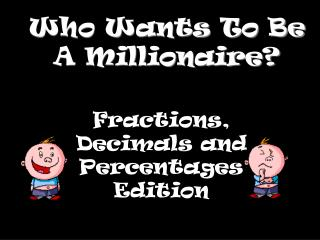DownloadDownload PresentationWho Wants To Be A Millionaire?

# Who Wants To Be A Millionaire?

Télécharger la présentation## Who Wants To Be A Millionaire?

- - - - - - - - - - - - - - - - - - - - - - - - - - - E N D - - - - - - - - - - - - - - - - - - - - - - - - - - -
##### Presentation Transcript

1. Who Wants To Be A Millionaire? Fractions, Decimals and Percentages Edition

2. Question 1

3. What is 10 % of 57? A 570 B 5 C 5.7 D 0.57

4. What is 10 % of 57? A 570 B 5 C 5.7 D 0.57.

5. £100

6. Question 2

7. What is the decimal equivalent of 62% A 62 B 0.062 C 0.62 D 6.2

8. What is the decimal equivalent of 62% A 62 B 0.062 C 0.62 D 6.2

9. £200

10. Question 3

11. What is 15 % of 85? A 8.5 B 12.75 C 72.75 D 12.25

12. What is 15 % of 85? A 8.5 B 12.75 C 72.75 D 12.25

13. £300

14. Question 4

15. Calculate 4/5 of 250 A 150 B 520 C 50 D 200

16. Calculate 4/5 of 250 A 150 B 520 C 50 D 200

17. £500

18. Question 5

19. Which of the following is greater than 4/7 of 42? A 20 B 6 C 28 D 21

20. Which of the following is greater than 4/7 of 42? A 20 B 6 C 28 D 21

21. £1,000

22. Question 6

23. Which of the following is less than 45 % of 180? A 90 B 88 C 85 D 80

24. Which of the following is less than 45 % of 180? A 90 B 88 C 85 D 80

25. £2,000

26. Question 7

27. How many percent is 3/5? A 60% B 30% C 50% D 40%

28. How many percent is 3/5? A 60% B 30% C 50% D 40%

29. £4,000

30. Question 8

31. What is 60% of 4 litres in ml? A 240ml B 2400ml C 24ml D 24000ml

32. What is 60% of 4 litres in ml? A 240ml B 2400ml C 24ml D 24000ml

33. £8,000

34. Question 9

35. If John has a bag of 30 sweets and he eats 21. What percentage is left? A 70% B 10% C 3% D 30%

36. If John has a bag of 30 sweets and he eats 21. What percentage is left? A 70% B 10% C 3% D 30%

37. £16,000

38. Question 10

39. Penguins leave 2/8 of the 120 fish that are thrown into their pool. How many fish do they eat? A 30 B 90 C 105 D 15

40. Penguins leave 2/8 of the 120 fish that are thrown into their pool. How many fish do they eat? A 30 B 90 C 105 D 15

41. £32,000

42. Question 11

43. What is four ninths of 72? A 8 B 23 C 32 D 49

44. What is four ninths of 72? A 8 B 23 C 32 D 49

45. £64,000

46. Question 12

47. Which of the following fractions are not equivalent to 80% ? A eight - tenths B four - fifths C eight - hundredths D eighty-hundredths

48. Which of the following fractions are not equivalent to 80% ? A eight - tenths B four - fifths C eight - hundredths D eighty-hundredths

49. £125,000

50. Question 13